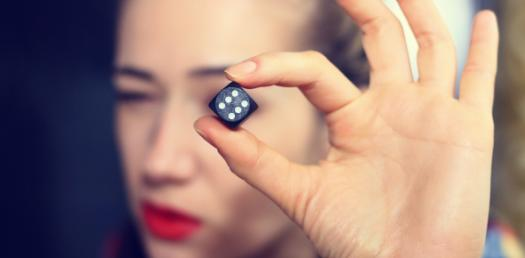# Quiz: Take The Basic Probability Test Questions!

16 Questions | Attempts: 6761SettingsCan you guess what kind of a score you would have you took a probability test? Define all of the possible events and outcomes that can occur if you roll dice, which has six sides and land on three. We know there are six possible events and one outcome. The number of possible outcomes is six. So take this quiz to see what you know.

• 1.
What is probability?
• A.

A way of counting objects in a space

• B.

A way of expressing knowledge or belief that an event will occur or has occurred

• C.

A way for people to predict if something will happen

• 2.
The menu at a restaurant has 3 drinks, 4 appetizers, and 5 main courses.  How many different meals are possible?
• A.

12

• B.

15

• C.

60

• 3.
On a game show, the game that a contestant plays is chosen by spinning a spinner.  The spinner has 5 colors on it:  red, green, blue, purple, and orange.   What is the complement of spinning a red or orange on this spinner? (Remember: complement = NOT)
• A.

Purple

• B.

Red, green, purple, orange, blue

• C.

Green, purple, blue

• 4.
John has a bag of marbles.  In the bag, there are 4 yellow marbles, 7 red marbles, and 3 green marbles.  What is the probability that John will pull out a yellow marble?
• 5.
When a die is rolled, what is the complement of getting an odd number?
• A.

Getting an even number

• B.

Getting an odd number

• C.

Getting a 4 or 5

• 6.
There are 20 girls and 35 boys in the lunch room.  What is the probability of choosing a boy to sit with?
• 7.
There are 52 cards in a deck.  What is the probability of choosing a jack?
• 8.
There are 10 pencils, 3 pens, and 14 markers in a bin.  What is the probability of choosing a pen and a pencil?
• A.

3/729

• B.

10/27

• C.

30/729

• D.

13/27

• 9.
There are 52 cards in a deck.  What is the probability of choosing a jack and a king?
• A.

16/2704

• B.

52/2704

• C.

4/52

• D.

8/52

• 10.
There are 34 kittens, 12 dogs, and 15 birds at a pet store.  What is the probability that a customer will purchase a dog?
• 11.
A coin is tossed.  What is the probability of getting heads?
• 12.
A die is rolled. What is the complement of rolling a 5?
• A.

Rolling a 1, 2, 3, 4, or 5

• B.

Rolling a 1, 2, 3, or 4

• C.

Rolling a 5

• 13.
There are 6 pink, 2 blue, and 8 green socks in a drawer.  What is the probability of the complement of picking a pink sock? (Remember: complement = NOT)
• A.

6/16

• B.

2/16

• C.

10/16

• 14.
Jason has a bag full of coins.  There are 12 dimes, 21 pennies, 13 nickels, and 7 quarters in his bag.  If he chooses a coin at random, what is the probability that he chooses either a quarter and a dime?
• A.

84/2809

• B.

12/53

• C.

19/2809

• D.

19/53

• 15.
Sarah has 4 tomatoes, 3 potatoes, and 5 apples in her bag.  What is the probability of the complement of her eating a tomato? (Remember: complement = NOT)
• A.

8/12

• B.

4/12

• C.

5/12

• 16.
Katherine has 7 shirts, 3 pairs of pants, and 4 pairs of socks.  How many different outfits can she make?
• A.

14

• B.

84

• C.

21

## Related TopicsBack to top
×

Wait!
Here's an interesting quiz for you.